Computer Science

# Combining Conditions

Let’s go back to the blue trail problem: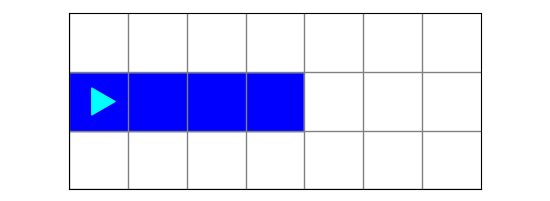What we want here is for Bit to keep going if:

• the front is clear, and
• the current square is blue

In another situation, we may want Bit to paint a square blue if:

• the current square is red, or
• the current square is green

Notice that we are combining conditions using the word and or the word or. We can do this in Python!

## and

When we use the keyword `and` to combine conditions, Python makes the following decision:

first conditionsecond conditionresult
TrueTrueTrue
TrueFalseFalse
FalseTrueFalse
FalseFalseFalse

In other words, the combination of two conditions with and means the result is `True` only if both of them are `True`. The combination is `False` if either one of the conditions is `False`.

If we want to keep going if the front is clear and the current square is blue, we could write a function like this:

``````def should_keep_going(bit):
if bit.front_clear() and bit.is_blue():
return True
else:
return False``````

These are the two conditions we are combining with `and`: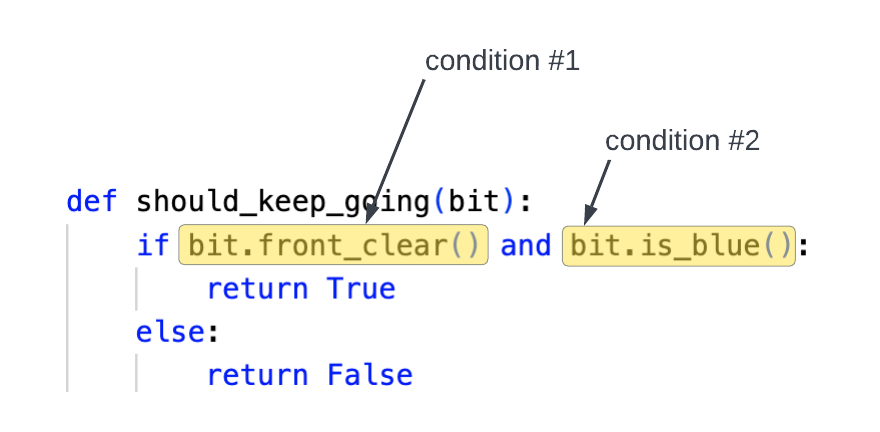### Shortcut

We can rewrite the above function using a shorter version:

``````def should_keep_going(bit):
return bit.front_clear() and bit.is_blue():``````

Any time `bit.front_clear()` and `bit.is_blue()` are both true, then this function will return `True`. Otherwise, it will return `False`. This is the same as the longer version above!

### Example

Let’s see this in action. Download the zip file linked above and store it in your `bit` folder. Find the file called `blue_trail.py`:

``````from byubit import Bit

def should_keep_going(bit):
return bit.front_clear() and bit.is_blue()

@Bit.worlds('blue_trail', 'blue_trail2')
def go(bit):
while should_keep_going(bit):
bit.move()

if __name__ == '__main__':
go(Bit.new_bit)``````

Run this code and you should verify it works the same as the longer version we wrote in the guide for return.

## or

When we use the keyword `or` to combine conditions, Python makes the following decision:

first conditionsecond conditionresult
TrueTrueTrue
TrueFalseTrue
FalseTrueTrue
FalseFalseFalse

In other words, the combination of two conditions with or means the result is `True` only if either of them is `True`. The combination is `False` only if both of the conditions are `False`.

If we want to paint a square blue if it is red or green, we could write a function like this:

``````def should_be_blue(bit):
return bit.is_red() or bit.is_green()``````

Notice that we are using a shortcut, with just a single return statement. This will return `True` if either the current square is red or if the current square is green.

### Example

Here is a Bit world with some red and green squares: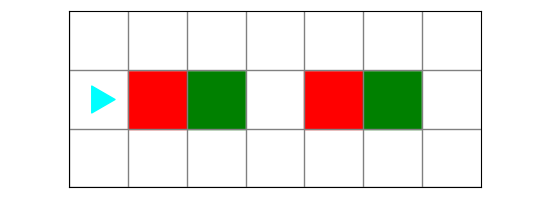Bit’s job is to paint a square blue if it is currently red or green. You can see code to do this by opening `red-or-green.py`:

``````from byubit import Bit

def should_be_blue(bit):
return bit.is_red() or bit.is_green()

@Bit.worlds('red-or-green')
def go(bit):
while bit.front_clear():
bit.move()
if should_be_blue(bit):
bit.paint('blue')

if __name__ == '__main__':
go(Bit.new_bit)``````

Run this code and step through it to see how it works. It finishes with this world: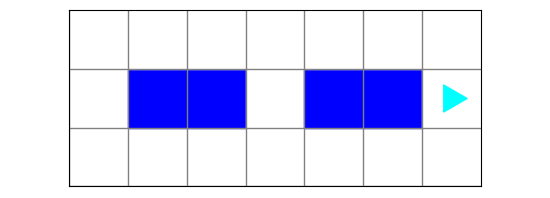## Crossing the pond

Let’s look at an example that adds `not` to go with `and` and `or`. Here is Bit’s world for this problem: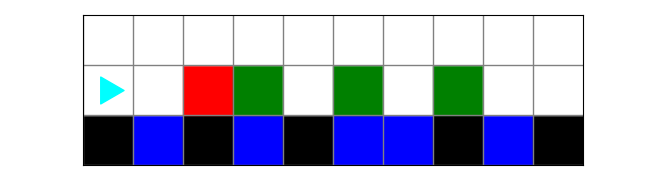• The green squares above blue are lily pads. They don’t jump away as Bit passes by.

• The red squares above black are flowers. They don’t jump either.

• The green squares above black are frogs. They will jump if Bit passes by.

We want to move Bit to the other side of the pond, making frogs jump away.

The ending world should look like this: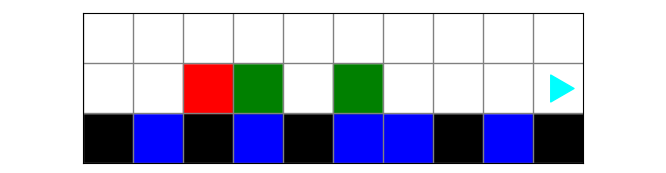In this problem, Bit needs to decide if the square it is on is a frog. Look at the rules above. We can’t just say a square is a frog if it is green, because some green squares are lily pads. A squares is a frog if it is green and the square below it is black. That means we could write this function:

``````def is_frog(bit):
"""Square is a frog if it is green and above a black square"""
return bit.is_green() and not bit.right_clear()``````

We use `bit.is_green() and not bit.right_clear()` to get the right condition. Notice it uses `and not` together.

To see this in action, open the file called `cross_the_pond.py`:

``````from byubit import Bit

def is_frog(bit):
"""Square is a frog if it is green and above a black square"""
return bit.is_green() and not bit.right_clear()

@Bit.worlds('frog-on-rock')
@Bit.pictures()
def go(bit):
while bit.front_clear():
bit.move()
if is_frog(bit):
bit.erase()

if __name__ == '__main__':
go(Bit.new_bit)
``````

Bit moves forward and if the current square is a frog, we erase that square, to indicate the frog has jumped away. We have put the logic for checking if the square represents a frog into the `is_frog()` function.